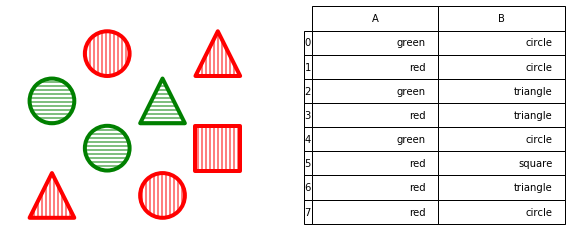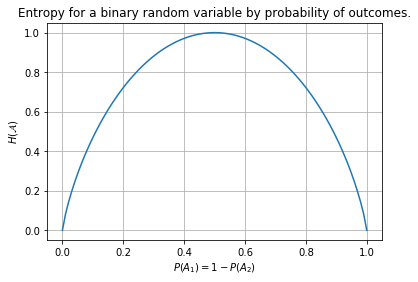# Understanding Conditional Entropy and Information Gain¶

## An Example¶

We have eight shapes in a bag that are either triangles, squares, or circles. Their color is either red or green.

In :
draw_obj(example_obj);In :
display_dfs(example, 'Dataset', proba, 'Joint and marginal distributions')

Dataset
A B
0 green circle
1 red circle
2 green triangle
3 red triangle
4 green circle
5 red square
6 red triangle
7 red circle
Joint and marginal distributions
$B_i$ circle square triangle $$P(Ai)$$
$A_i$
green 0.25 0 0.125 0.375
red 0.25 0.125 0.25 0.625
$$P(Bi)$$ 0.5 0.125 0.375 1

In the second table, the six cells top left show the joint probability distribution $P(A_i,B_i)$. The final column and final row show the marginal probability distributions for $\mathcal{A}$ and $\mathcal{B}$, respectively.

## Entropy¶

Recall from the lecture:

Let $A$ denote an event and let $P(A)$ denote the occurrence probability of $A$. Then the entropy (self-information, information content) of $A$ is defined as $-\kern-2pt\log{_2}(P(A))$.

Let $\mathcal{A}$ be an experiment with the exclusive outcomes (events) $A_1,\ldots,A_k$. Then the mean information content of $\mathcal{A}$, denoted as $H(\mathcal{A})$, is called Shannon entropy or entropy of experiment $\mathcal{A}$ and is defined as follows:

$H(\mathcal{A}) = -\sum_{i=1}^k P(A_i) \cdot \log_2(P(A_i))$

In other words, the Shannon entropy gives a us measure for the degree of "surprise" from learning the value of $\mathcal{A}$.

In :
display_dfs(example, 'Dataset', proba, 'Joint and marginal distributions'); entropy_calculation(example, 'A')

Dataset
A B
0 green circle
1 red circle
2 green triangle
3 red triangle
4 green circle
5 red square
6 red triangle
7 red circle
Joint and marginal distributions
$B_i$ circle square triangle $$P(Ai)$$
$A_i$
green 0.25 0 0.125 0.375
red 0.25 0.125 0.25 0.625
$$P(Bi)$$ 0.5 0.125 0.375 1
Out:
\begin{align}H(\mathcal{ A }) &= - \left(P(\mathcal{ A }=green) \cdot \log_2 P(\mathcal{ A }=green)~+~P(\mathcal{ A }=red) \cdot \log_2 P(\mathcal{ A }=red)\right) \\ &= - \left(0.375\cdot\log_2 0.375~+~0.625\cdot\log_2 0.625\right) \quad = \quad 0.954 \end{align}

For a binary variable, the entropy reaches a maximum of 1.0 when both outcomes are equally probable.

In other words, an entropy of 1 bit corresponds to the information content of a (fair) coin toss.

In :
binary_entropy()In :
display_dfs(example, 'Dataset', proba, 'Joint and marginal probabilities', '\n'); entropy_calculation(example, 'A', 'B')

Dataset
A B
0 green circle
1 red circle
2 green triangle
3 red triangle
4 green circle
5 red square
6 red triangle
7 red circle
Joint and marginal probabilities
$B_i$ circle square triangle $$P(Ai)$$
$A_i$
green 0.25 0 0.125 0.375
red 0.25 0.125 0.25 0.625
$$P(Bi)$$ 0.5 0.125 0.375 1
Out:
\begin{align}H(\mathcal{ A }) &= - \left(P(\mathcal{ A }=green) \cdot \log_2 P(\mathcal{ A }=green)~+~P(\mathcal{ A }=red) \cdot \log_2 P(\mathcal{ A }=red)\right) \\ &= - \left(0.375\cdot\log_2 0.375~+~0.625\cdot\log_2 0.625\right) \quad = \quad 0.954 \\H(\mathcal{ B }) &= - \left(P(\mathcal{ B }=circle) \cdot \log_2 P(\mathcal{ B }=circle)~+~P(\mathcal{ B }=triangle) \cdot \log_2 P(\mathcal{ B }=triangle)~+~P(\mathcal{ B }=square) \cdot \log_2 P(\mathcal{ B }=square)\right) \\ &= - \left(0.500\cdot\log_2 0.500~+~0.375\cdot\log_2 0.375~+~0.125\cdot\log_2 0.125\right) \quad = \quad 1.406 \end{align}

## Conditional Entropy¶

We now want to answer the question, how much information about variable A is still uncertain, after we learn the value of variable B?

Recall from the slides:

Let $\mathcal{A}$ be an experiment with the exclusive outcomes (events) $A_1,\ldots,A_k$, and let $\mathcal{B}$ be another experiment with the outcomes $B_1,\ldots,B_s$. Then the conditional entropy of the combined experiment $(\mathcal{A}\mid\mathcal{B})$ is defined as follows:

$H(\mathcal{A}\mid\mathcal{B}) = \sum_{j=1}^s P(B_j) \cdot {H(\mathcal{A}\mid B_j)}$

where

${H(\mathcal{A}\ {\mid B_j})} = - \sum_{i=1}^k P(A_i\ {\color{\textcolorB}\mid B_j}) \cdot\log{_2}(P(A_i\ {\color{\textcolorB}\mid B_j}))$

In :
display_dfs(example, 'Dataset', proba, 'Joint and marginal distributions', entropies, 'Entropies', '\n', cp, 'Conditional probabilities $P(A_i | B_i)$', ch, 'Specific Conditional entropies');

Dataset
A B
0 green circle
1 red circle
2 green triangle
3 red triangle
4 green circle
5 red square
6 red triangle
7 red circle
Joint and marginal distributions
$B_i$ circle square triangle $$P(Ai)$$
$A_i$
green 0.25 0 0.125 0.375
red 0.25 0.125 0.25 0.625
$$P(Bi)$$ 0.5 0.125 0.375 1
Entropies
entropy
variable
$$H(\mathcal{A})$$ 0.954
$$H(\mathcal{B})$$ 1.406
Conditional probabilities $P(A_i | B_i)$
$B_i$ circle square triangle
$A_i$
green 0.5 0 0.333
red 0.5 1 0.667
Specific Conditional entropies
$${H(\mathcal{A}|B_i)}$$
$B_i$
circle 1
square 0
triangle 0.918

#### Specific Conditional Entropy¶

The expression $H(\mathcal{A}\mid B_i)$ reads as "the entropy of variable $\mathcal{A}$ among only those records that have $\mathcal{B}=B_i$."

In our example, if we know that $\mathcal{B}=\mathrm{'circle'}$, the value of $\mathcal{A}$ is exactly as uncertain as a coin toss, but not so for the other possible values of $\mathcal{B}$.

#### Conditional Entropy¶

The expression $H(\mathcal{A}|\mathcal{B})$ is the average of $H(\mathcal{A}|B_i)$ over all possible values of $\mathcal{B}$, each weighted by their probability.

In :
display_dfs(proba, 'Joint and marginal distributions', cp, 'Conditional probabilities $P(A_i | B_i)$', ch, 'Specific Conditional entropies');
calc_cond_ent(ch, proba, "A", "B")

Joint and marginal distributions
$B_i$ circle square triangle $$P(Ai)$$
$A_i$
green 0.25 0 0.125 0.375
red 0.25 0.125 0.25 0.625
$$P(Bi)$$ 0.5 0.125 0.375 1
Conditional probabilities $P(A_i | B_i)$
$B_i$ circle square triangle
$A_i$
green 0.5 0 0.333
red 0.5 1 0.667
Specific Conditional entropies
$${H(\mathcal{A}|B_i)}$$
$B_i$
circle 1
square 0
triangle 0.918
Out:
\begin{align} \ H(\mathcal{ A }\mid \mathcal{ B }) &= \sum_{j=1}^s P(B_j)\cdot H(\mathcal{ A }\mid B_j) \\ &= P(circle) \cdot H(\mathcal{A}\mid circle)~+~P(square) \cdot H(\mathcal{A}\mid square)~+~P(triangle) \cdot H(\mathcal{A}\mid triangle) \\ &= 0.5 \cdot 1~+~0.125 \cdot 0~+~0.375 \cdot 0.918 \\ &= 0.844 \end{align}

# Information Gain¶

In :
display_dfs(entropies, 'Entropies');
calc_cond_ent(ch, proba, "A", "B")

Entropies
entropy
variable
$$H(\mathcal{A})$$ 0.954
$$H(\mathcal{B})$$ 1.406
Out:
\begin{align} \ H(\mathcal{ A }\mid \mathcal{ B }) &= \sum_{j=1}^s P(B_j)\cdot H(\mathcal{ A }\mid B_j) \\ &= P(circle) \cdot H(\mathcal{A}\mid circle)~+~P(square) \cdot H(\mathcal{A}\mid square)~+~P(triangle) \cdot H(\mathcal{A}\mid triangle) \\ &= 0.5 \cdot 1~+~0.125 \cdot 0~+~0.375 \cdot 0.918 \\ &= 0.844 \end{align}

The information gain tells us how much we learn about variable $\mathcal{A}$ by knowing the value of variable $\mathcal{B}$, expressed as the reduction in entropy:

\begin{align} H(\mathcal{A}) - H(\mathcal{A}\mid\mathcal{B}) &= 0.954 - 0.844 = 0.11 \end{align}